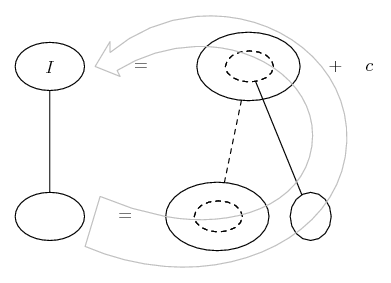# Thread: 2 question calculus questions!

1. ## 2 question calculus questions!

1.) what exactly is u substitution? is it the chain rule backwards??

2.) when u right in function in terms of y instead of x, does the graph physically change? if not what's the purpose in doing so? also is it possible to have my calculator work with a function in terms of y?

2. 1) A u sub usually just makes the problem easier to solve rather than being necessary in it's own right.

For example which is easier to solve? $\displaystyle \displaystyle \int \dfrac{\sin(x)}{cos^2(x)}\, dx$ or $\displaystyle \displaystyle \int \dfrac{du}{u^2}$

I would say the "chain rule backwards" is when you have a integral of the kind $\displaystyle \int \dfrac{u'(x)}{u(x)} dx$

2) I don't understand what you mean but from what I understand it would be a reflection in the line $\displaystyle y=x$ (as per inverse functions)

3.Originally Posted by maybnxtseasn1.) what exactly is u substitution? is it the chain rule backwards??
Absolutely, no question. E to i pi is right to point out that we often don't bother with a u-sub if the integrand is transparently the result of a chain rule differentiation, but when we do use a u-sub its purpose is to work backwards through the chain rule despite its possibly being hidden. And it does no harm to think of it that way. E.g...and... where (key in spoiler) ...

Spoiler:... is the chain rule. Straight continuous lines differentiate downwards (integrate up) with respect to the main variable (in this case x), and the straight dashed line similarly but with respect to the dashed balloon expression (the inner function of the composite which is subject to the chain rule).

The general drift is...PS: and what is integration by parts a matter of working backwards through...?As for your question 2, the graph will physically change (reflect in the line y=x as e to i pi says) if y is necessarily the vertical and x horizontal, but this isn't always so. The horizontal can be x, y, t or whatever.

_________________________________________

Don't integrate - balloontegrate!

Balloon Calculus; standard integrals, derivatives and methods

Balloon Calculus Drawing with LaTeX and Asymptote!

#### Search Tags

calculus, question, questions